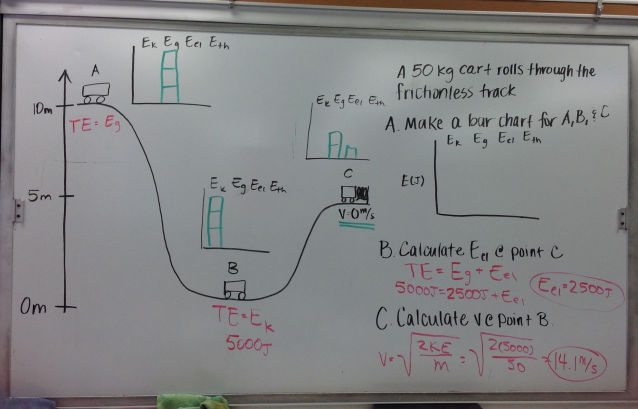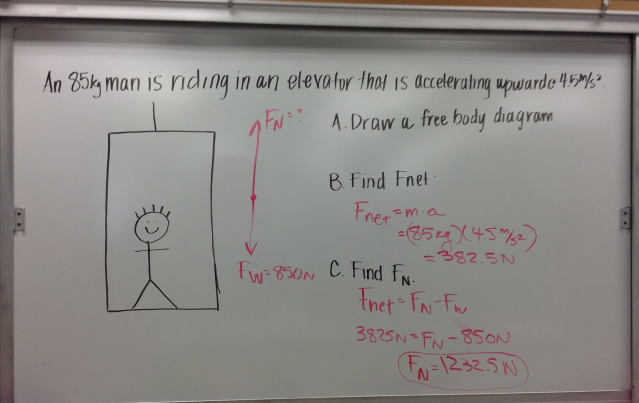# Physics Review: Inner/Outer Circle Activity

1 teachers like this lesson
Print Lesson

## Objective

Students will be able to choose the correct equation to solve problems from various units throughout the semester.

#### Big Idea

Students practice calculations from all seven units including motion, Newton's Laws, energy, waves & electrostatics.

## Energy Conservation Practice Problem

10 minutes

The goal of this lesson is to help students to review the computational, problem-based aspects of the semester. Students practice many different types of calculations from various units and decide what equation is appropriate for each problem based on what unit it comes from. This is a true test of their computational thinking (SP5) as they complete the problems and really have decide what equations are appropriate for the problem they are currently working on.

To start out class, I give students another practice problem similar to the previous class, as shown in the image below. I choose this energy conservation problem because it is among the list of concepts that students told me that they wanted to practice before the final exam. The problem combines many aspects of the energy unit including topics of qualitative conservation of energy (bar charts) and quantitative conservation of energy (finding elastic energy and velocity). This relates to the energy unit that students learned about previously and relates directly to HS-PS4-1 and HS-PS4-3.I give students about 5 minutes to work on this individually and then I go over it as a class. I ask students to volunteer for each part and make sure to call on a different student for each part to get many students involved in this review. As I go over this problem, I emphasize that finding elastic energy is similar to how we found kinetic energy in the roller coaster problems; I just asked for something a little different this time. Finally, I make sure students recognize that the total energy equals the potential energy at the highest point and the kinetic energy at the lowest point.

## Physics Final Inner/Outer Circle Review Activity

50 minutes

After students have finished the practice problem. I tell students we are going to complete an Final Inner/Outer Circle Review and have students explain what they do throughout the activity. Each student receives a card with a problem on it that also has the answer on the back. Each student then solves the problem and checks his or her answer to the answer on the back. Since the answers are on the back I expect my students to show all of their work.

Before I pass out the problem cards, I pass out a blank sheet of paper to each student. I ask them to fold it in half, the "hot dog" way. Then I ask them to unfold it and fold it twice the "hamburger" way so that when unfolded there are 8 boxes, as shown below:Then I ask them to put their name in 1 box. After their papers are folded, I pass out a problem to each student. The problems are labeled as numbers and letters that help to organize students later in the activity. There are easy, medium and hard questions randomly throughout the lettered and numbered problem cards. I make sure to give each student a problem that is the appropriate level of difficulty based on how they have been doing in class.

Once each student has a problem I tell the students where to sit. I have A and 1 sit at the same table across from each other. Similarly, I have B and 2, C and 3, etc. sit across from each other. When all students are sitting across from their partner, I tell them that each pair has a similar problem in which they use the same equation. Each student needs to complete his or her own problem, but if he or she needs help or gets the wrong answer he or she can ask the partner to help. I give pairs 2.5 minutes to complete their own problem. Then I remind them that they are now experts on their problem and carry their problem with them throughout the activity. I also make sure that they take their Final Equation Card with them that has all of the equations from all of the units that they need.

After the 2.5 minutes, I ask the lettered card holders to stay seated and the numbers to rotate to the next table in a circle. When they get to their new partner, I ask them to switch cards with their partner and do their partner's problem. If they need help, they can ask the person sitting across from them because this person is now an expert on that problem. Students get 2.5 minutes to complete the new problem. When they are done, they switch problems so they are holding their original problem. Then the numbers once again rotate to the next table. Students continue to rotate every 2.5 minutes until the end of the period. A student's work is shown below.As students work through the problems, I walk around to monitor their progress and to keep them focused on their work. I use this activity because it holds students accountable for explaining their thought processes to other students. It also allows students to complete problems using various equations. I like this activity because students rely on each other and also get a lot of practice with problems for an entire period.

## Newton's Second Law Elevator Practice Problem

10 minutes

To end class, I have students complete an elevator problem using Newton's 2nd Law, as shown in the image below. I wait to do this problem until after the students complete the inner/outer circle activity so they can practice it once before we do another practice problem on the board. Several students ask specifically for me to go over the elevator problem before we finish reviewing for final exams. I give students about 5 minutes to work on this problem on their own and then we go over it as a class. I try to call on as many students as possible to go over this problem. I emphasize that doing all 3 parts in this order is very important to solving this problem. This problem is related to the content from the Force and Acceleration Unit.This is the second of the three review lessons. The purpose of this lesson is to help students to review the computational aspects of all of the units which is what the inner/outer circle review activity does for students.